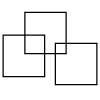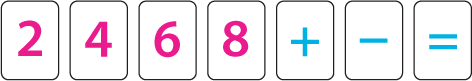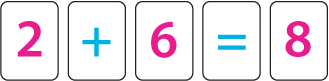#### You may also like### Three Squares

What is the greatest number of squares you can make by overlapping three squares?### Two Dice

Find all the numbers that can be made by adding the dots on two dice.### Biscuit Decorations

Andrew decorated 20 biscuits to take to a party. He lined them up and put icing on every second biscuit and different decorations on other biscuits. How many biscuits weren't decorated?

# 2,4,6,8

## 2, 4, 6, 8

Use these cards to make calculations with their answers,Like this one:In each calculation you must only use a card once, but of course you can re-use them in your next calculation if you want to.

Can you find a way to use all the cards in one calculation?

Can you find all the possible ways to use the cards?

### Why do this problem?

This problem gives children the opportunity to create their own number sentences. The discussion it provokes will give them the opportunity to use vocabulary related to addition and subtraction, and to begin to develop ways of working systematically.

### Possible approach

Having the digits and operation signs on the interactive whiteboard will allow you flexibility to be led by the children as they tackle this task. This very simple interactivity could be useful. You could begin by dragging some of the numbers and operations to make an incorrect number sentence. Invite children to talk in pairs about what is on the board. Ask learners to share their thoughts with everyone which will no doubt mean that your error is pointed out. How could they make the sum correct? Invite a pair to do this alongside the incorrect number sentence so that the original version can still be seen. Ask if another pair can make it right in a different way. Collect some other suggestions and then set up the first task. Working with these number and operation cards, pairs can try out their ideas.

Gather the class together to talk about this first challenge and then introduce the second one so that this time they don't have to use all the cards, but must make as many true number statements as they can. As they work, encourage them to record what they have done, and look out of those that have a system of some description, for example finding all the sentences with $8$ to being with, then with $6$ etc.

You could ask children to write each sentence on a separate strip of paper so in the plenary they could put these up on a wall. That way, you can ask them to identify ones that "go together" (for example all those which start with $8$ and are subtractions). By moving them around to make groups, the children will be able to identify any that are missing so you can highlight the advantage of ordering solutions in this way. A rich discussion can be had to decide whether, for example, $2 + 4 = 6$ is the same as $4 + 2 = 6$.

### Key questions

What have you tried so far?
How will you know you have found all the different number sentences?
Are there any other sums that have the $8$ in them?
Are there any other addition sums?
Can you make any number sentences with all four numbers?

### Possible extension

Children could be encouraged to consider a similar problem using the odd digits less than ten. Does this create difficulties? If so, why?
The set of numbers being considered can be extended in various ways. The children can choose and be encouraged to think about extensions which generate interesting results and those that do not do so.
A similar idea could be introduced using multiplication and division as well as addition and subtraction.

### Possible support

Some children may need the support of  concrete apparatus such as counters or Multilink cubes to make the calculations. trying out some one step calculations using these digits would be a good introductory task.
You could break the task down a bit for some children, for example, by asking them to find all the number sentences which use the $2$ first, then the $4$ etc. Having these cards to manipulate will mean that children will find it easier to change their mind rather than feeling pressured to having a correct calculation immediately.